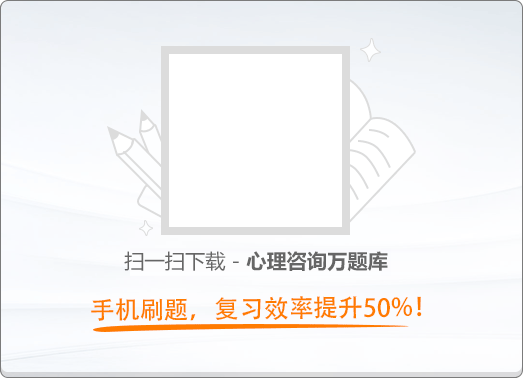90:002016年05月二级心理咨询师《职业道德、理论知识》真题

1
(单项选择题)

• A.

职业是责任、权利、利益的有机统一

• B.

职业是人们生存的唯一手段

• C.

职业是束缚人的只有、权利的锁链

• D.

职业是赚取人们剩余劳动价值的方式

• A
• B
• C
• D

2
(单项选择题)

• A.

集体主义

• B.

为人民服务

• C.

爱国主义

• D.

科学发展观

• A
• B
• C
• D

3
(单项选择题)

• A.

忠诚、谨慎、进取

• B.

忠诚、敬业、诚信

• C.

诚信、手法、人本

• D.

忠诚、审慎、勤勉

• A
• B
• C
• D

4
(单项选择题)

• A.

站在自己的立场上为客户着想

• B.

要把奖金和薪水作为最强大的工作动力

• C.

对公司的产品具有寻根究底的好奇心

• D.

通过自己的成功，帮助老板成功

• A
• B
• C
• D

5
(单项选择题)

• A.

相互信任，同时也反对不明是非、不分善恶的盲目信任

• B.

既信任，又怀疑

• C.

表面上信任，实际上不信任

• D.

即使信任，也要加强防范和戒备

• A
• B
• C
• D

6
(单项选择题)

• A.

经营者的职工采用商业贿赂手段为经营者购销商品，认定为职工个人行为

• B.

在账外暗中给予对方单位或者个人回扣的，以行贿论处

• C.

任何经营者购销产品，均不可以明示方式给中间人佣金

• D.

经营者在商品交易中不得向对方单位附赠现金或者物品，包括小额广告礼品

• A
• B
• C
• D

7
(单项选择题)

• A.

点子不重要，执行力重要

• B.

战略问题不重要，策略问题重要

• C.

执行、落实和纪律比没有实际行动的空想重要

• D.

点子靠别人，执行靠自己

• A
• B
• C
• D

8
(单项选择题)

• A.

在节约问题上，不可能形成统一的价值观念

• B.

节约因人而异，企业节约主要是上司的责任

• C.

花多少钱，办多少事，只要不超支便是节约

• D.

节约方式虽有差异，但节约精神具有共通性

• A
• B
• C
• D

9
(多项选择题)

• A.

爱国守法

• B.

诚实守信

• C.

自强创新

• D.

奉献社会

• A
• B
• C
• D

10
(多项选择题)

• A.

职业化包含三个层次的内容，其中核心层是职业化素养

• B.

职业化也称为“专业化”，它是一种自律性的工作态度

• C.

职业化要求从业人员在工作和决策中尽量发挥主观性和个人兴趣

• D.

职业化在行为标准方面的体现称为职业化行为规范

• A
• B
• C
• D

11
(多项选择题)

• A.

尊敬、尊崇自己的职业

• B.

对待工作恭敬、虔诚

• C.

享受工作的过程

• D.

精益求精，不断进步

• A
• B
• C
• D

12
(多项选择题)

• A.

发自内心，不自欺欺人

• B.

承诺的力度要量力而行

• C.

有“承”必践，有“约”必履

• D.

充分相信他人的承诺

• A
• B
• C
• D

13
(多项选择题)

• A.

自然万物的客观实在性

• B.

对“天道”的真实反映

• C.

尊重事实和忠实本心的待人对物的态度

• D.

从辞源上看，就是“把事情说成了”

• A
• B
• C
• D

14
(多项选择题)

• A.

择业信用

• B.

岗位责任信用

• C.

家庭信用

• D.

离职信用

• A
• B
• C
• D

15
(多项选择题)

• A.

企业供热系统的运行、管理和余热利用，应当按国家有关规定执行

• B.

企业供用电的技术要求，按照国家标准局的有关规定执行

• C.

企业应编制节能改造的中长期规划和年度计划

• D.

企业使用国家已公布淘汰的机电产品和超能耗标准设备，须停用或转移

• A
• B
• C
• D

16
(多项选择题)

• A.

求同存异

• B.

互助协作

• C.

公平竞争

• D.

不拘小节

• A
• B
• C
• D